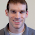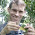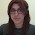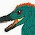## Monday, April 11, 2011

### New function: phyl.pca()

I just posted a new function for phylogenetic principal components analysis (as described in my 2009 paper, here). In my article, I gave R code to do this as an appendix; however, this new function expands on that originally very simple function by eliminating some preliminaries (e.g., the operation vcv.phylo(...), for instance), as well as by adding the estimation of joint lambda (by way of method="lambda") for the variables in our input matrix.

The function is available on my R-phylogenetics page (direct link to code here).

To use, just load the function from source:

> source("phyl.pca.R")

then type:

> result<-phyl.pca(tree,X,method="lambda",mode="corr")

(or some variant thereof, depending on the settings desired.)

Unfortunately, likelihood estimation using method="lambda" is pretty slow. I have tried to speed it up a little by minimizing the number of calls to solve(...) and use of the matrix operator %*%. Note that optimization time here scaled with the number of taxa × the number of traits (rather than just with n, as in phyl.resid()).

I did this a couple of different ways. First, I simply avoided multiple calls to solve(C) whenever possible by first setting invC<-solve(C). Unfortunately, optimization of lambda using likelihood requires constant readjustment of C, so this probably had pretty limited impact. Second, I substituted the calculation sum(invC) for the matrix operation 1'C-11 and colSums(invC%*%X) for 1'C-1X.

At some point I will try to update this using functions from the package {Matrix} which I'm told are considerably faster than the matrix operators in R {base}. When I get around to this I will report the result!

For more details on this analysis - I refer the reader to my paper.

#### 17 comments:

1.Hi Liam,

First, I enjoy reading your posts!

I just wanted to point out that in most practical situations, one does not need to invert matrices. For example, in the above function, what you really want is matrix inverse times some vector. It is much faster to accomplish this task with solve() directly than first getting the inverse and then multiplying it by a vector.

A simple demonstration is below:

C = matrix(rnorm(10000),100,100);
a = rnorm(100);

system.time(for (i in 1:1000) solve(C)%*%a);
system.time(for (i in 1:1000) solve(C,a));

2.Thanks Vladimir.

I have basically been using solve(...) to invert, rather than to "solve." Computing C^-1*Y is the same as solving Y=CX for X; except that solve(C,Y) is faster than solve(C)%*%Y, as you point out.

I will note, though, that once I have computed invC<-solve(C), *then* invC%*%X becomes considerably faster than solve(C,X). So I guess it depends how many times I would normally invert C.

After some reflection, though, I think the biggest inefficiency of my code is the repeated and unnecessary calls of vcv.phylo(). I will try and fix both of these issues and then I will post an updated version (along with the comparison).

3.Liam,

It's probably a moot point anyway, since you found a more important inefficiency in your code, but from your original post it seems that it makes sense to cach 1'C^-1 rather than C^-1. This of course makes sense only if you are always multiplying C^-1 by the same vector from the left, but use different vectors for right multiplication. Demonstration is below:

C = matrix(rnorm(10000),100,100);
a = rnorm(100);
x = rep(1,100);

system.time(for (i in 1:1000){A=solve(C); x%*%A%*%a; x%*%A%*%x});
system.time(for (i in 1:1000){A=solve(C); colSums(A%*%a); sum(A)});
system.time(for (i in 1:1000){A=solve(t(C),x); A%*%a; A%*%x});

On my machine, this gives more than 2 fold speed up.

4.When you have two vectors that you use to multiply C^-1 from the left, the speed up is not that impressive, only ~20%:

system.time(for (i in 1:1000){A=solve(C); x%*%A%*%a; x%*%A%*%x; a%*%A%*%x; a%*%A%*%x;});
system.time(for (i in 1:1000){A=solve(t(C),x); A%*%a; A%*%x; A=solve(t(C),a); A%*%a; A%*%x});

5.Hi Vladimir.

You pre-empted my "improvements" post here.

The biggest issue is that the comparison is really:

system.time(A<-solve(C); for(i in 1:1000) A%*%B)
# vs.
system.time(for(i in 1:1000) solve(C,B))

That said, however, I did successfully swap solve(A,B) for solve(A)%*%B in one place in my code that it made a huge difference; that is for the likelihood calculation involving a n*m x n*m Kronecker product matrix. Here, on a sample 100 species tree with 10 traits, I saved about 0.75s per calculation by following your suggestion! This is fantastic since this calculation will be performed many times during ML optimization.

Thanks!

6.Would it be simple enough to create a nonmetric multidimensional scaling version of this function? Instead of using PCA that is.

7.Hi Scott.
I don't know that this would be simple.
What would be our goal in incorporating phylogenetic information into, say, classical MDS? I'd imagine it would be to minimize the influence of correlated distances between close relatives. Has this been attempted in any study, to your knowledge?
Thanks for the comment.

8.Hi Liam,

I have used ordination for species traits, and we used MDS (although we didn't compare to results of PCA). From my understanding, MDS avoids assuming linear relationships among variables (traits could be related in a nonlinear fashion), contrary to PCA I think. Might this be a good reason to use MDS instad of PCA for species traits? Of course transformation of traits could linearize them sufficiently.

My thought was that you can use MDS axes just as you would use PCA axes.

I haven't been able to find any attempts of phylogenetic MDS in the literature yet.

9.Hi Scott.
I think in that case we would want to compute MDS axes to minimize the influence of distances that are correlated due to the phylogeny. This should be possible so long as we have a way to get the expected distances between related species on a phylogeny given some model of evolution (say, BM).

10.Hi Liam,

Thanks for the updated phyl.pca function. The code in the direct link doesn't run as written, but it looks like a small error. The trait matrix variable is initially named Y, but then switches to X after the line # analyze. I just changed Y to X and now it runs fine.

11.Hmmm. This (in theory) should not be the problem, as the "change" to X is inside internal functions called by phyl.pca(). Still, that doesn't explain why changing it to be X throughout resolved the issue! I will investigate this.

12.Hello Liam. I'm currently using tpsRelw as I know exactly how it handles sliding semi landmarks, yet still wish to perform your phyl.pca. I think the best way to do this would be to obtain instead my partial warps (as opposed to relative warps, the normal final step in tpsRelw) from tps Relw, and then subject this matrix (of taxa and scores) to your function. Can you see any issue with this?

1.Hi John. Although this seems reasonable, I'm afraid I don't know enough about geometric morphometrics to comment on this. There may be complicated issues that I don't appreciate. Why don't you try R-sig-phylo with this question? Good luck. - Liam

2.Dear John,
Perhaps I can be of assistance to you. The phylogenetic PCA is not yet implemented in the Geometric Morphometrics packages for R (such as Geomorph, Morpho or Shapes). Before proceeding, I suggest reading a little more on this ordination method in Polly et al. 2013 Hystrix and Monterio 2013 Hystrix (http://www.italian-journal-of-mammalogy.it/public/journals/3/issue_241_complete_100.pdf). Leandro Monterio has his own R code for including the phylogenetic covariance matrix during a PCA of landmark data, and David Polly has his Mathematica programs. Dean Adams, Erik Otárola-Castillo and I have no immediate plans to implement the phylo PCA into Geomorph (http://cran.r-project.org/web/packages/geomorph/), although you can put in a formal request to Dr. Adams. You can also contact the Morphmet list to see if anyone else has already worked up some R code.
Best wishes,
Emma

3.Thank you both very much for your thoughts, I'm looking into this.

13.Hi Liam, I+ve trying to use this function, but I do not have a traits matrix (and I am actually not really sure about what it is). Could you give me some insight? Thanks

1.Traits would just be the Y argument, a matrix with columns as your variables of interest with rows as your taxa.

Note: due to the very large amount of spam, all comments are now automatically submitted for moderation.